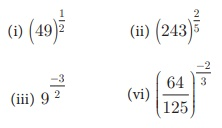Home | | Maths 9th std | Exercise 2.5: Radical Notation

## Chapter: 9th Maths : UNIT 2 : Real Numbers

Maths : Real Numbers : Book Back, Exercise, Example Numerical Question with Answers, Solution : Numerical Problems with Answers, Solution : Exercise 2.5: Radical Notation

Exercise 2.5

1. Write the following in the form of 5n :

(i) 625 (ii) 1/5 (iii) √5  (iv) √1252. Write the following in the form of 4n:

(i) 16 (ii) 8 (iii) 323. Find the value of4. Use a fractional index to write:

(i) √5 (ii) 2√7 (iii) (3√49)5 (iv)5. Find the 5th root of

(i) 32 (ii) 243 (iii) 100000 (iv) 1024/ 3125Tags : Numerical Problems with Answers, Solution | Real Numbers | Maths , 9th Maths : UNIT 2 : Real Numbers
Study Material, Lecturing Notes, Assignment, Reference, Wiki description explanation, brief detail
9th Maths : UNIT 2 : Real Numbers : Exercise 2.5: Radical Notation | Numerical Problems with Answers, Solution | Real Numbers | Maths